Math & Beyond

# Embedded Figures

14th Aug '200 views7 mins read

 1 What are Embedded Figures? 2 What is Embedded Figures Test? 3 How the Embedded Figures Test is Helpful for Kids? 4 Understanding of Embedded Figures 5 Identify a Small Part Hidden in the Given Figure 6 Embedded Figures Test 7 Summary

14 August 2020

## What are Embedded Figures?

Embedded figures are the figures hidden inside the other figure.

• In other words, a figure (X) is said to be embedded inside figure (Y), if figure (Y) contains a part of Figure (X).
• We are required to select from the alternatives that figure clearly shows the embedded portion of the original figures.

Examples: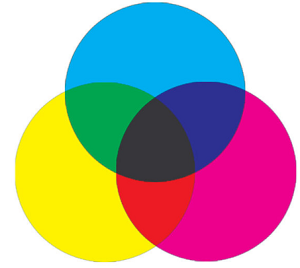## What is Embedded Figures Test?

Embedded Figures Test is a test that consists of identifying a simple form embedded within a complex figure. This test can also be used to look at analytical style, social behaviors and problem solving style.

### Steps to Solve

• Do not get tensed by seeing the complexity of the figures.
• Carefully understand the form of the given question figure.
• Sometimes the rotation or reflection of the figures may be confusing.
• Think wisely before selecting the answer.

## How the Embedded Figures Test is Helpful for Kids?

Embedded Figures test is designed to see how your child can use critical thinking and approach to solve problems, and are an indication of their mathematical capabilities and powers of deduction
Embedded Figures Tests are used to look at analytical style, social behaviors and problem solving style.

• Embedded figures are a very easy topic and questions from this are asked in Olympiad and other examinations to test the child's attention to detail.
• Practicing these questions regularly will improve the child’s logical reasoning skills and Embedded figure reasoning.

## Understanding of Embedded Figures

Embedded figure questions consist of a unique figure which is hidden in one of the given option figures. In such questions, all the options look similar and confusing. So children need to understand clearly while answering such questions. Anyhow with a good practice children can master these Non-Verbal figures. We must basically understand the form of the given question figure and then proceed to find the correct option figure.
Below are the few examples with solutions for better understanding of Embedded Figures.

### Example 1:

Question: Observe the figure carefully and answer the question.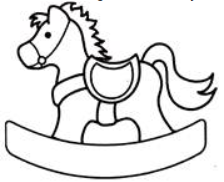Which part is hidden/embedded in above figure?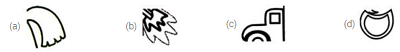Step 1: Option a’s figure is not hidden/embedded in the given figure
Step 2: Option b’s figure is not hidden/embedded in the given figure
Step 3: Option c’s figure is not hidden/embedded in the given figure
Step 4: Option d’s figure is hidden/embedded in the given figure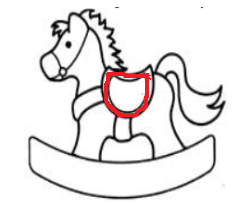Out of four options only option ‘d’ (figure) is embedded in the given figure. So the answer is (d).

### Example 2:

Question: Which of the following parts is hidden/embedded in the given figure (X)?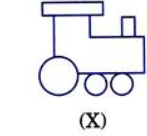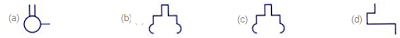Step 1: Option a’s figure is not hidden/embedded in the given figure
Step 2: Option b’s figure is not hidden/embedded in the given figure
Step 3: Option c’s figure is not hidden/embedded in the given figure
Step 4: Option d’s figure is embedded in the given figure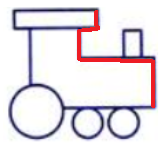Out of four options only option ‘d’ (figure) is embedded in the given figure. So the answer is (d).

## Identify a Small Part Hidden in the Given Figure

A figure (X) is given, followed by options (parts of a given figure)  such that one of them is embedded in the given figure (X). We need  to identify such parts. Below are the few examples to Embedded part of figure problems

### Example 1

Question: In the given question, find the figure from the options which is hidden/embedded in Figure X.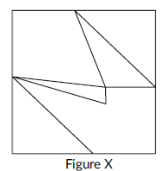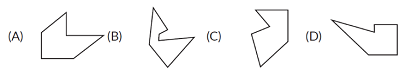Step 1: Option a’s figure is not hidden/embedded in the given figure
Step 2: Option b’s figure is not hidden/embedded in the given figure
Step 3: Option c’s figure is not hidden/embedded in the given figure
Step 4: Option d’s figure is hidden/embedded in the given figure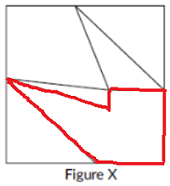Out of four options only option ‘d’ (figure) is embedded in the given figure. So the answer is (d).

### Example 2

Question: In the given question, find the figure from the options which is hidden/embedded in Figure X.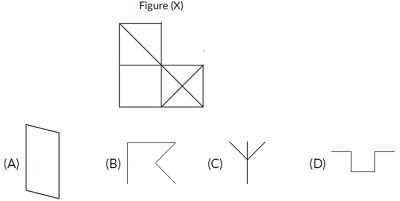Step 1: Option a’s figure is not hidden/embedded in the given figure.
Step 2: Option b’s figure is hidden/embedded in the given figure.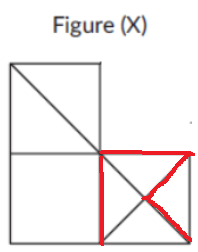Out of four options only option ‘b’ (figure) is embedded in the given figure. So the answer is (b).

## Identify the Figure in which the Given Part is Hidden

In these types of problems, a figure (X) is followed by given complex figures in such a way that figure (X) is embedded in one and only one of them. we have to select such a figure in which figure (X) is hidden/embedded.

Below are the few examples to Identify a small part hidden in the given figure

### Example 1

Question: Identify a small part hidden in the given figure.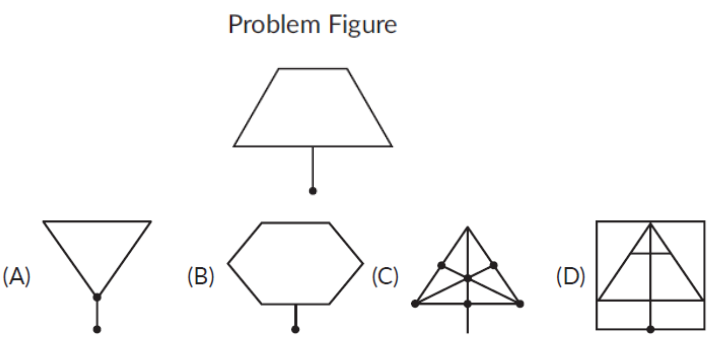Step 1: Option a’s figure is not hidden/embedded in the given figure.
Step 2: Option b’s figure is not hidden/embedded in the given figure.
Step 3: Option C’s figure is hidden/embedded in the given figure.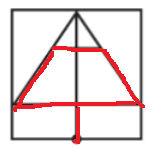Out of four options only option ‘C’ (figure) is embedded in the given figure. So the answer is C.

### Example 2

Question: Which of the four options has parts that exactly match the figure given here?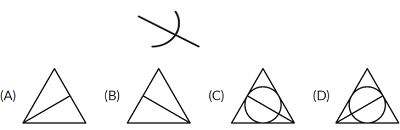Step 1: Option a’s figure is not hidden/embedded in the given figure.
Step 2: Option b’s figure is not hidden/embedded in the given figure.
Step 3: Option C’s figure is hidden/embedded in the given figure.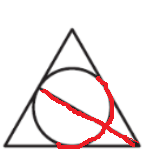Out of four options only option ‘C’ (figure) is embedded in the given figure. So the answer is (C).

## Embedded Figures Test

Question: Which of the four options exactly matches a part of the figure here?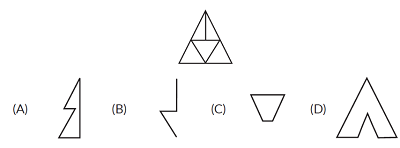Question: Which of the four options exactly matches a part of the figure here?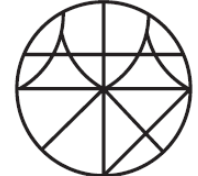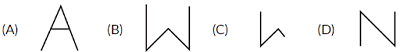Question: Which option has the given shape exactly embedded without any rotation as one of its parts?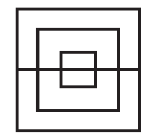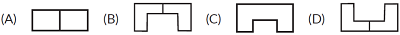Question: Which of the four options has part that exactly matches the figure here?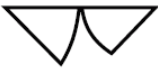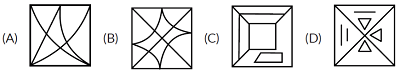Question: Which of the four options has part that exactly matches the figure here?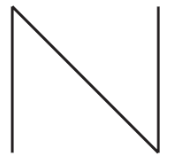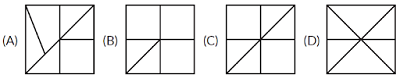Question: Which of the four options has part that exactly matches the figure here?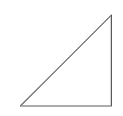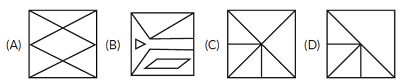## Summary

• Embedded figures are the figures hidden inside the other figure.
• Embedded Figures Tests are used to look at analytical style, social behaviors and problem solving approach.
• Types of embedded figures:
1. Identify a small part hidden in the given figure
2. Identify the figure in which the given part is hidden.
• Embedded Figures Test is a test that consists of identifying and drawing a simple form embedded within a complex figure.

Related Articles
GIVE YOUR CHILD THE CUEMATH EDGE
Access Personalised Math learning through interactive worksheets, gamified concepts and grade-wise courses